How Cheenta works to ensure student success?
Explore the Back-Story

# Test of Mathematics Solution Subjective 188 - The Numbered Chessboard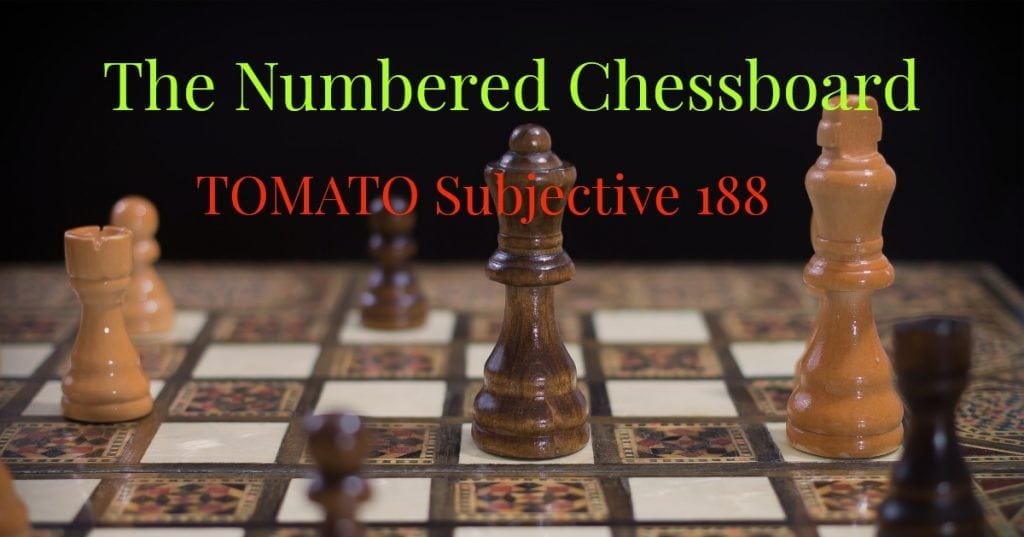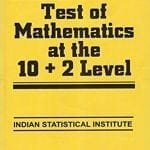This is a Test of Mathematics Solution Subjective 188 (from ISI Entrance). The book, Test of Mathematics at 10+2 Level is Published by East West Press. This problem book is indispensable for the preparation of I.S.I. B.Stat and B.Math Entrance.

## Problem

Consider the squares of an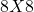chessboard filled with the numbers 1 to 64 as in the figure below. If we choose 8 squares with the property that there is exactly one from each row and exactly one from each column, and add up the numbers in the chosen squares, show that the sum always adds up to 260.## Solution

The problem asks us to choose numbers selectively such that they are from unique rows and columns. We write the numbers in the table in such a manner that helps us in our calculations. This is how it will be done:

Let the number in therow and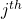column be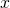. If we carefully observe the table we find an intuitive way of representingas follows: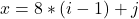ifis the element in therow andcolumn. Now all that is left to do is sum up all such numbers such that no two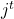orvalue is same. There for the total sum is: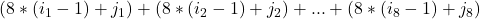that means Sum (S) =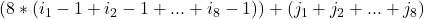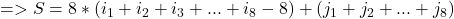We know that as all theand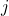values are different and range from 1 to 8 we can ascertain that

the sum of thevaluessum ofvalues =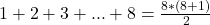Therefore S =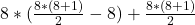=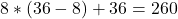Hence the sum of all the values taken from unique rows and columns is 260.

Hence Proved.

## Chatuspathi:

• What is this topic: Arithmetic
• What are some of the associated concept: Invariance.
• Where can learn these topics: Cheenta I.S.I. & C.M.I. course, discusses these topics in the ‘Arithmetic’ module.
• Book Suggestions: Mathematical Circles by Fomin.This is a Test of Mathematics Solution Subjective 188 (from ISI Entrance). The book, Test of Mathematics at 10+2 Level is Published by East West Press. This problem book is indispensable for the preparation of I.S.I. B.Stat and B.Math Entrance.

## Problem

Consider the squares of anchessboard filled with the numbers 1 to 64 as in the figure below. If we choose 8 squares with the property that there is exactly one from each row and exactly one from each column, and add up the numbers in the chosen squares, show that the sum always adds up to 260.## Solution

The problem asks us to choose numbers selectively such that they are from unique rows and columns. We write the numbers in the table in such a manner that helps us in our calculations. This is how it will be done:

Let the number in therow andcolumn be. If we carefully observe the table we find an intuitive way of representingas follows:ifis the element in therow andcolumn. Now all that is left to do is sum up all such numbers such that no twoorvalue is same. There for the total sum is:that means Sum (S) =We know that as all theandvalues are different and range from 1 to 8 we can ascertain that

the sum of thevaluessum ofvalues =Therefore S ==Hence the sum of all the values taken from unique rows and columns is 260.

Hence Proved.

## Chatuspathi:

• What is this topic: Arithmetic
• What are some of the associated concept: Invariance.
• Where can learn these topics: Cheenta I.S.I. & C.M.I. course, discusses these topics in the ‘Arithmetic’ module.
• Book Suggestions: Mathematical Circles by Fomin.

### Knowledge Partner